### Calculating data in rows and columns

Using PerfectExpert, you can calculate data in rows and columns. For example, you can add a column, find the average of a list of figures, or find the middle value of either.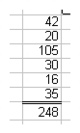This is an example of the Quick Math feature automatically entering a formula into a cell to calculate a column of values.

You can also perform calculations on individual cells.

Quattro Pro also lets you perform calculations on two rows or columns.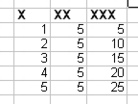In this example, the cell in last row of the first column is multiplied by the cell in the last row of the second column and the total is displayed in the cell in the last row of the third column.

#### To calculate data in a single row or column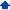1 Click Help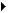PerfectExpert.
 2 Click Do simple math in the PerfectExpert panel.
 3 Click Quick math.
 4 In the Select a row or column box, specify the cells to calculate.
 5 Choose a math operation from the Do math list box.
 6 In the Insert answer in cell box, specify the cell where you want to display the answer.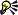• You can also specify cells by clicking the Range picker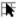tool and selecting the cells.

#### To perform a calculation on each item in a row or column1 Click HelpPerfectExpert.
 2 Click Do simple math in the PerfectExpert panel.
 3 Click More quick math.
 4 In the Select a row or column box, specify the cells to calculate.
 5 Choose a math operation from the Do math list box.
 6 In the Insert answer in cell box, specify the cell where you want to display the answer.
 The answer row or column must have as many cells as are in the list on which you’re performing calculations.• You can also specify cells by clicking the Range pickertool and selecting the cells.

#### To calculate data in two rows or columns1 Click HelpPerfectExpert.
 2 Click Do simple math in the PerfectExpert panel.
 3 Click 2-column quick math.
 4 In the Select first row/column box, specify the cells to calculate for the first row or column.
 5 In the Select second row/column box, specify the cells to calculate for the second row or column.
 6 Choose a math operation from the Do math list box.
 7 In the Insert answer in cell box, specify the cell where you want to display the answer.
 The answer row or column must have as many cells as are in the list on which you’re performing calculations.• You can also specify cells by clicking the Range pickertool and selecting the cells.